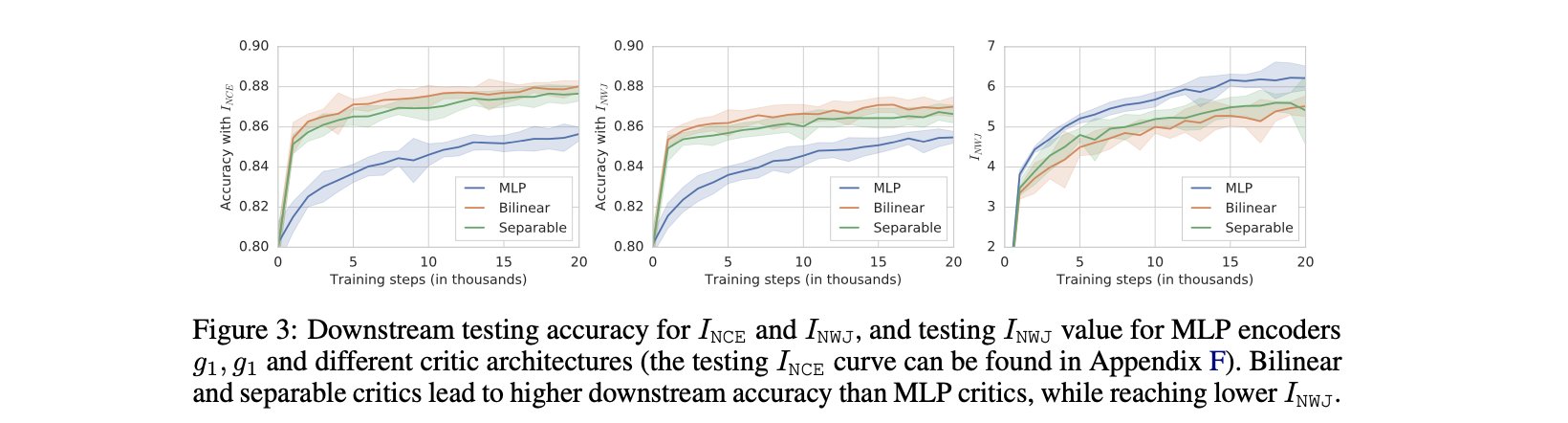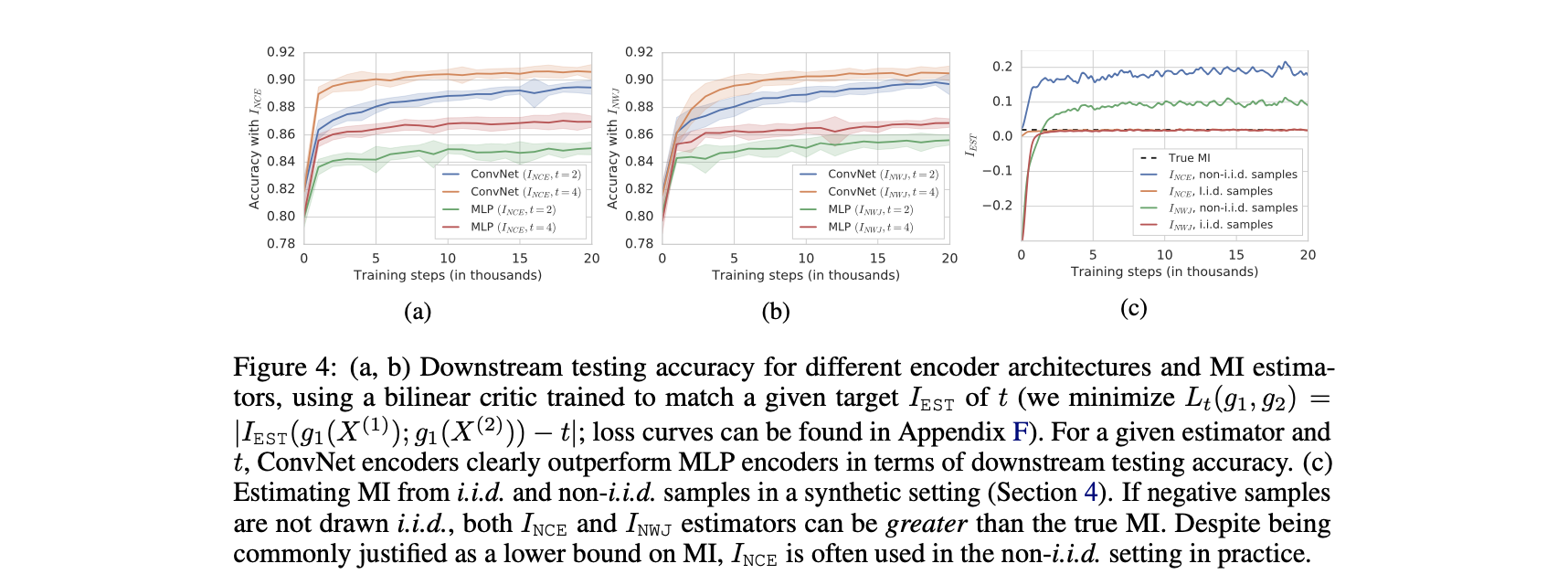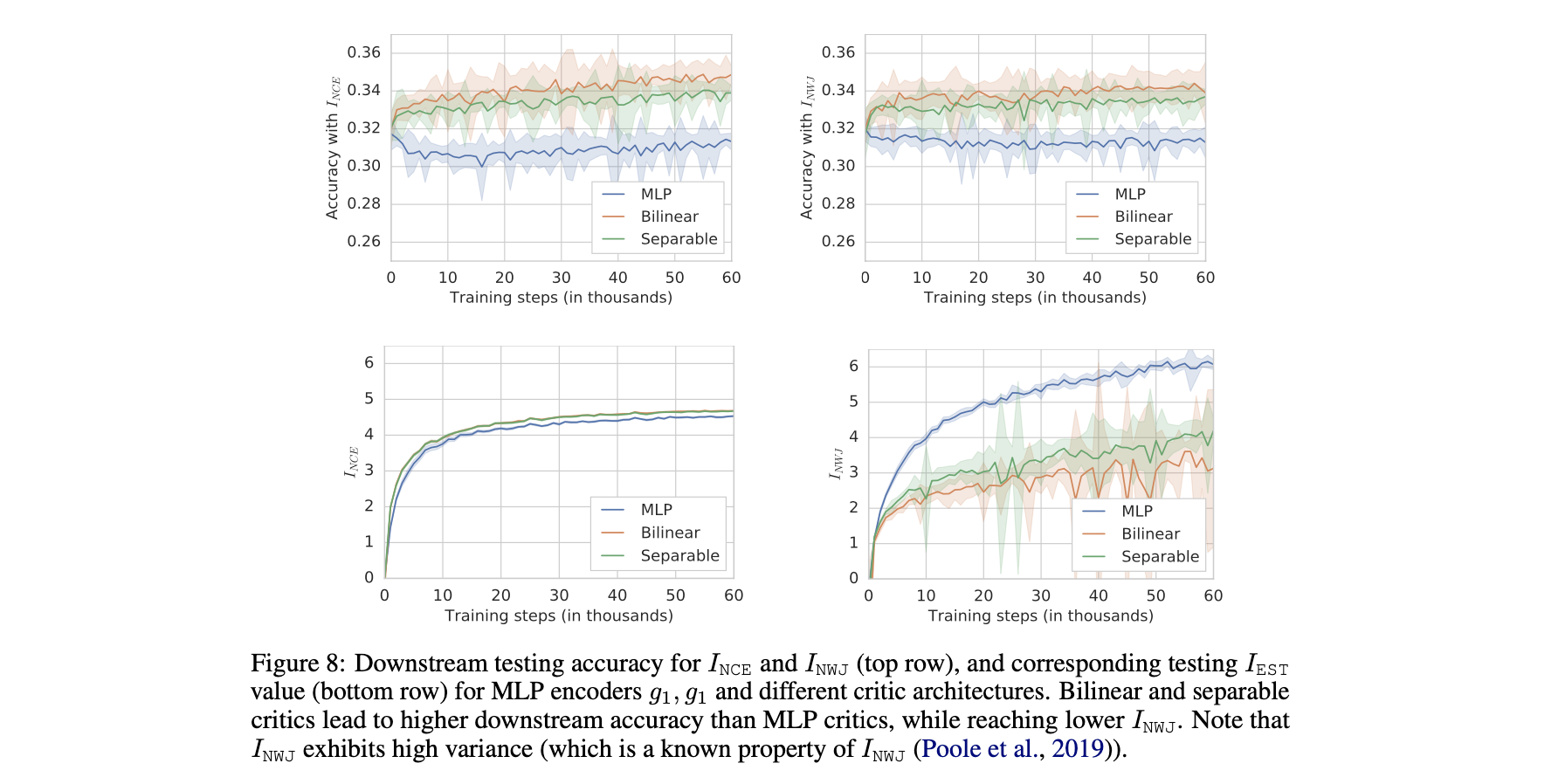N1H111SM's Miniverse

# On MI Maximization for Representation Learning

2020/05/22 ShareMaterials

# Motivation

In this paper we argue, and provide empirical evidence, that the success of these methods cannot be attributed to the properties of MI alone, and that they strongly depend on the inductive bias in both the choice of feature extractor architectures and the parametrization of the employed MI estimators.

## Structure of Introduction

• What is MI: definition
• Fundamental properties of MI
• Firstly, MI is invariant under reparametrization of the variables - namely, if $X^\prime = f_1(X)$ and $Y^\prime = f_2(Y)$ are homeomorphisms (i.e. smooth invertible maps), then $I (X ; Y ) = I (X ^\prime ; Y ^\prime )$.
• Secondly, estimating MI in high-dimensional spaces is a notoriously difficult task, and in practice one often maximizes a tractable lower bound on this quantity.
• Any distribution-free high-confidence lower bound on entropy requires a sample size exponential in the size of the bound.
• In fact, we show that maximizing tighter bounds on MI can result in worse representations.
• In addition, we establish a connection to deep metric learning and argue that this interpretation may be a plausible explanation of the success of the recently introduced methods.

## Multi-view formulation of the MI maximization

In the image classification setting, for a given image $X$, let $X^{(1)}$ and $X^{(2)}$ be different, possibly overlapping views of $X$, for instance the top and bottom halves of the image. These are encoded using encoders $g_1$ and $g_2$ respectively, and the MI between the two representationsg $g_1(X^{(1)})$ and $g_2(X^{(2)})$ is maximized,

where $\hat I$ represents the sample based MI estimator of the true MI $I(X;Y)$ and the function classes $\mathcal{G}_{1}$ and $\mathcal{G}_{2}$ can be used to specify structural constraints on the encoders. One thing to note here is that two encoders $g_1$ and $g_2$ often share parameters.

It gives us plenty of modeling flexibility, as the two views can be chosen to capture completely different aspects and modalities of the data, for example:

• In the basic form of DeepInfoMax $g_1$ extracts global features from the entire image $X^{(1)}$ and $g_2$ local features from image patches $X^{(2)}$, where $g_1$ and $g_2$ correspond to activations in different layers of the same convolutional network. (也就是上一节介绍的global/local-MI Maximization)
• Contrastive multiview coding (CMC) generalizes the objective in to consider multiple views $X^{(i)}$, where each $X^{(i)}$ corresponds to a different image modality (e.g., different color channels, or the image and its segmentation mask).
• Contrastive predictive coding(CPC) incorporates a sequential component of the data. Concretely, one extracts a sequence of patches from an image in some fixed order, maps each patch using an encoder, aggregates the resulting features of the first $t$ patches into a context vector, and maximizes the MI between the context and features extracted from the patch at position $t+k$

# Biases in Approximate MI Maximization

• Encoder: influenced by network structures / parameters.
• Critics: the function tells between the
• Estimators: the lower bound to optimize.

• encoders are bijective. (not reported in this blog)
• encoders that can model both invertible and non-invertible functions. (not reported in this blog)
• different critics: simple / loose bound would lead to high-capacity critics.
• encoder is more important than architectures.

## Setup

Motivation: Our goal is to provide a minimal set of easily reproducible empirical experiments to understand the role of MI estimators, critic and encoder architectures when learning representations via the objective.

Dataset: To this end, we consider a simple setup of learning a representation of the top half of MNIST handwritten digit images. Try to maximize the mutual information of the representation between the representation of the top half and the bottom half of the images. Using bilinear critic: $f(x,y)=x^TWy$.

Evaluation: Following the widely adopted downstream linear evaluation protocol.

## Higher Capacity Critics: Worse Downstream Tasks

In the previous section we have established that MI and downstream performance are only loosely connected. Clearly, maximizing MI is not sufficient to learn good representations and there is a non-trivial interplay between the architectures of the encoder, critic, and the underlying estimators.

Compared 3 different architectures of the critic functions:

• a bilinear critic,
• a separable critic $f(x,y) = \phi_1(x)^⊤\phi_2(y)$ ($\phi_1$, $\phi_2$ are MLPs with a single hidden layer with 100 units and ReLU activations, followed by a linear layer with 100 units; comprising 40k parameters in total)
• an MLP critic with a single hidden layer with 200 units and ReLU activations, applied to the concatenated input [x, y] (40k trainable parameters).## Encoder Architecture: More Important than Specific Estimator

To ensure that both network architectures achieve the same lower bound $I_{EST}$ on the MI, we minimize $L_{t}\left(g_{1}, g_{2}\right)=| I_{\mathrm{EST}}\left(g_{1}\left(X^{(1)}\right) ; g_{1}\left(X^{(2)}\right)\right)-t |$ instead of solving original information maximization problem, for two different values $t = 2, 4$.# Deep Metric Learning

Given sets of triplets, namely an anchor point $x$, a positive instance $y$, and a negative instance $z$, the goal is to learn a representation $g(x)$ such that the distances between $g(x)$ and $g(y)$ is smaller than the distance between $g(x)$ and $g(z)$, for each triplet. 从这个角度也就不难看出为什么会称MI maximization中的函数为critic function. 而InfoNCE又可以被写作：

# Future Work

### Alternative measures of information

While MI has appealing theoretical properties, it is clearly not sufficient for this task—it is hard to estimate, invariant to bijections and can result in suboptimal representations which do not correlate with downstream performance. Therefore, a new notion of information should account for both the amount of information stored in a representation and the geometry of the induced space necessary for good performance on downstream tasks. One possible avenue is to consider extensions to MI which explicitly account for the modeling power and computational constraints of the observer, such as the recently introduced F-information.

### Going beyond the widely used linear evaluation protocol

While it was shown that learning good representations under the linear evaluation protocol can lead to reduced sample complexity for downstream tasks (Arora et al., 2019), some recent works (Bachman et al., 2019; Tian et al., 2019) report marginal improvements in terms of the downstream performance under a non-linear regime. Related to the previous point, it would hence be interesting to further explore the implications of the evaluation protocol, in particular its importance in the context of other design choices. We stress that a highly-nonlinear evaluation framework may result in better downstream performance, but it defeats the purpose of learning efficiently transferable data representations.